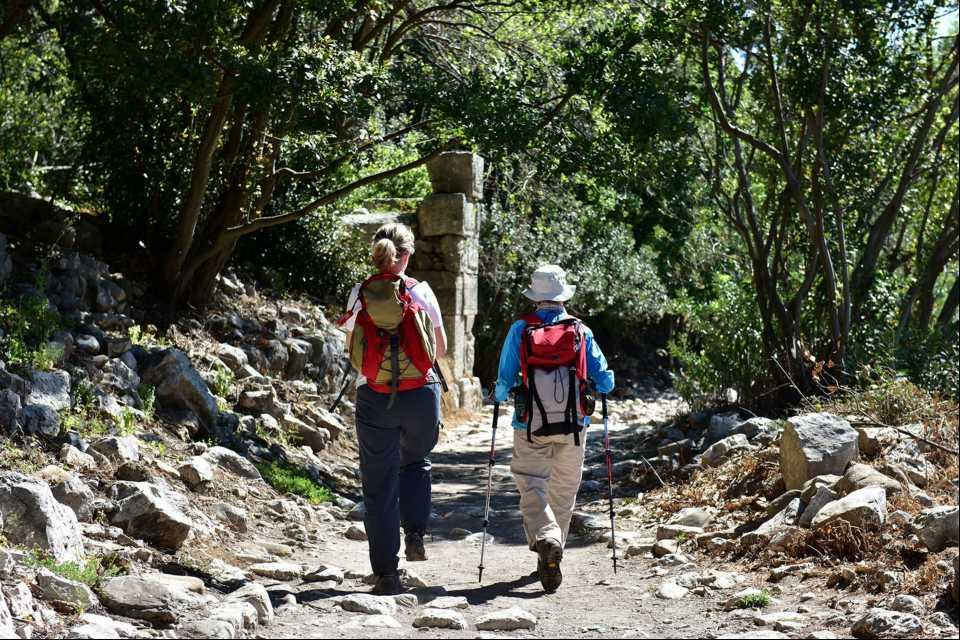< class="sp-layer sp-black sp-padding" data-position="topCenter" data-horizontal="0" data-vertical="50" data-show-transition="right" data-show-delay="500">< class="sp-layer sp-black sp-padding" data-position="topCenter" data-horizontal="0" data-vertical="50" data-show-transition="right" data-show-delay="500">< class="sp-layer sp-black sp-padding" data-position="topCenter" data-horizontal="0" data-vertical="50" data-show-transition="right" data-show-delay="500">< class="sp-layer sp-black sp-padding" data-position="topCenter" data-horizontal="0" data-vertical="50" data-show-transition="right" data-show-delay="500">< class="sp-layer sp-black sp-padding" data-position="topCenter" data-horizontal="0" data-vertical="50" data-show-transition="right" data-show-delay="500">< class="sp-layer sp-black sp-padding" data-position="topCenter" data-horizontal="0" data-vertical="50" data-show-transition="right" data-show-delay="500">< class="sp-layer sp-black sp-padding" data-position="topCenter" data-horizontal="0" data-vertical="50" data-show-transition="right" data-show-delay="500">< class="sp-layer sp-black sp-padding" data-position="topCenter" data-horizontal="0" data-vertical="50" data-show-transition="right" data-show-delay="500">< class="sp-layer sp-black sp-padding" data-position="topCenter" data-horizontal="0" data-vertical="50" data-show-transition="right" data-show-delay="500">< class="sp-layer sp-black sp-padding" data-position="topCenter" data-horizontal="0" data-vertical="50" data-show-transition="right" data-show-delay="500">< class="sp-layer sp-black sp-padding" data-position="topCenter" data-horizontal="0" data-vertical="50" data-show-transition="right" data-show-delay="500">< class="sp-layer sp-black sp-padding" data-position="topCenter" data-horizontal="0" data-vertical="50" data-show-transition="right" data-show-delay="500">< class="sp-layer sp-black sp-padding" data-position="topCenter" data-horizontal="0" data-vertical="50" data-show-transition="right" data-show-delay="500">< class="sp-layer sp-black sp-padding" data-position="topCenter" data-horizontal="0" data-vertical="50" data-show-transition="right" data-show-delay="500">< class="sp-layer sp-black sp-padding" data-position="topCenter" data-horizontal="0" data-vertical="50" data-show-transition="right" data-show-delay="500">< class="sp-layer sp-black sp-padding" data-position="topCenter" data-horizontal="0" data-vertical="50" data-show-transition="right" data-show-delay="500">< class="sp-layer sp-black sp-padding" data-position="topCenter" data-horizontal="0" data-vertical="50" data-show-transition="right" data-show-delay="500">< class="sp-layer sp-black sp-padding" data-position="topCenter" data-horizontal="0" data-vertical="50" data-show-transition="right" data-show-delay="500">< class="sp-layer sp-black sp-padding" data-position="topCenter" data-horizontal="0" data-vertical="50" data-show-transition="right" data-show-delay="500">< class="sp-layer sp-black sp-padding" data-position="topCenter" data-horizontal="0" data-vertical="50" data-show-transition="right" data-show-delay="500">< class="sp-layer sp-black sp-padding" data-position="topCenter" data-horizontal="0" data-vertical="50" data-show-transition="right" data-show-delay="500">< class="sp-layer sp-black sp-padding" data-position="topCenter" data-horizontal="0" data-vertical="50" data-show-transition="right" data-show-delay="500">< class="sp-layer sp-black sp-padding" data-position="topCenter" data-horizontal="0" data-vertical="50" data-show-transition="right" data-show-delay="500">< class="sp-layer sp-black sp-padding" data-position="topCenter" data-horizontal="0" data-vertical="50" data-show-transition="right" data-show-delay="500">< class="sp-layer sp-black sp-padding" data-position="topCenter" data-horizontal="0" data-vertical="50" data-show-transition="right" data-show-delay="500">< class="sp-layer sp-black sp-padding" data-position="topCenter" data-horizontal="0" data-vertical="50" data-show-transition="right" data-show-delay="500">< class="sp-layer sp-black sp-padding" data-position="topCenter" data-horizontal="0" data-vertical="50" data-show-transition="right" data-show-delay="500">< class="sp-layer sp-black sp-padding" data-position="topCenter" data-horizontal="0" data-vertical="50" data-show-transition="right" data-show-delay="500">< class="sp-layer sp-black sp-padding" data-position="topCenter" data-horizontal="0" data-vertical="50" data-show-transition="right" data-show-delay="500">< class="sp-layer sp-black sp-padding" data-position="topCenter" data-horizontal="0" data-vertical="50" data-show-transition="right" data-show-delay="500">< class="sp-layer sp-black sp-padding" data-position="topCenter" data-horizontal="0" data-vertical="50" data-show-transition="right" data-show-delay="500">< class="sp-layer sp-black sp-padding" data-position="topCenter" data-horizontal="0" data-vertical="50" data-show-transition="right" data-show-delay="500">Tour-Nr
:
LW 11
Duration
:
8 Days
Persons
:
1
Difficulty
:
B
Location
:
Antalya

Invalid Input
Invalid Input
Invalid email address form
Invalid input
Invalid Input
Invalid Input
ANTALYA WEATHER